### Common Errors in Secondary Mathematics

Common Errors Committed  by the  Students  in Secondary Mathematics   Errors  that students often make in doing secondary mathematics  during their practice and during the examinations  and their remedial measures are well explained here stp by step.  Some Common Errors in Mathematics

### Math Assignment Ch-10 Class X | Tangent to a circle

Mathematics Assignment Class X
Tangent to a Circle  Chapter 10
Maths assignment chapter 10 of class X. Maths assignment on tangent to the circle class 10th. Extra questions important for the CBSE examination for class X chapter 10.

 Question 1:  In the given figure the common tangents AB and CD to two circles intersect at E. Prove that AB = CDQuestion 2:  PT and PQ are two tangents from external point P. If ∠PQT = 70o, Find ∠x [Ans 20o]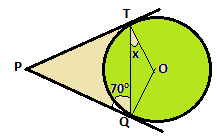Question 3AB is the chord of the circle with centre O, AOC is the diameter and AT is the tangent at A.Prove that . ∠1 = ∠2 Question 4PQ and PR are tangents, SR ∥ PQ,  ∠P = 30o then,  Find ∠x        [Ans 30o]Hint: ∠RQP = ∠PRQ  = 75o∠RQP = ∠S =  75o    (∵ Angles in the alternate segments are equal)∠S + ∠x + ∠RQP = 180oQuestion 5CP, CQ and AB are three tangents to the circle. If CP = 11 cm, BR = 4 cm. Thena) Find BC  [Ans 7 cm]b) Prove that : CP = (1/2) ✕ Perimeter of  ABCc) Find the perimeter of the   ABC  [Ans 22 cm]d) Prove that : CA + AR = CB + BRe) Prove that : AB = AP + BQf) If AC = 4 cm, AB = 5 cm, BC = 6 cm, then find CP  [Ans  7.5 cm]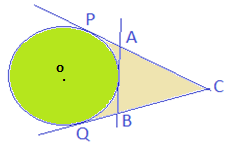Question 6For the given figure answer the following questionsa) If AB = 10 cm, AR = 7 cm, and RC = 5 cm, find BC [Ans 8 cm]b) If AB = 8 cm, BC = 10 cm, CA = 12 cm, then find AP, BQ and CR [Ans 7 cm, 5 cm, 3 cm]c) If AB = 8 cm, BC = 7 cm, CA = 5 cm, find BQ [Ans 5 cm]d) If AB = 8 cm, BC = 7 cm , CA = 5 cm, find the radius of the incircle [Ans √3]e) If AB = AC then prove that BQ = CQQuestion 7In △OPQ, OP =OQ = PQ = 6 cm, then find angle ∠PTQQuestion 8In △ABC, AC = 8 cm, AB = 6 cm, ∠A = 90o, find the radius of the circle.[Ans 2cm]Question 9PQ is the chord of length 6 cm of a circle of radius 6 cm, TP and TQ are tangents to the circle at point P and Q respectively. Find ∠PTQ. Also find the length TP.     Ans [120o , TP = √12 cm]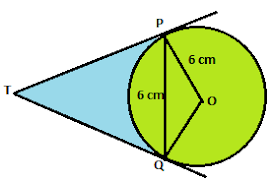Question 10AP and BP are tangents. OA = 8 cm and OB = 5 cm. If AP = 15 cm, then find the length of BP.  [Ans √264]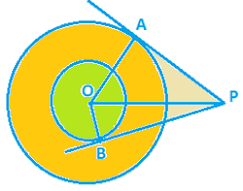Question 11In the given figure find the length of AB and ACAns [AB = 7.5, AC = 6.5 cm]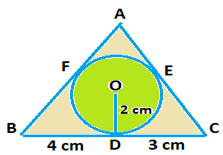Question 12∠D = 90o , BC = 38 cm, CD = 25 cm, and BP = 27 cm, find radius r of the circle. [Ans 14 cm]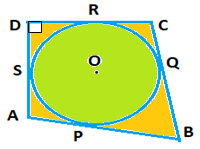Question 13O is the centre of the circle of radius 5 cm. T is a point such that OT = 13 cm and OT intersect the circle at E. If AB is the tangent to the circle at E, then find the length AB  [Ans 20/3]Hint :-        AT2  =  AE2 + ET2           (PT - AE)2  =  AE2  + ET2             (12 - AE)2 = AE2 + 82Question 14A chord PQ of a circle is parallel to the tangent drawn at a point R of the circle. Prove that point R bisects the arc PRQ.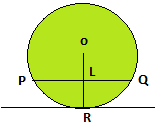Question 15The lengths of three consecutive sides of a quadrilateral circumscribing a circle are 4 cm, 5 cm, and 7 cm respectively. Find the length of the fourth side. [Ans 6 cm]Hint: x + y = 4 ….(i) Y + z = 5 …(ii) a + z = 7 ….(iii)Adding (i), (ii), (iii) we get: x + 2y + 2z + a = 16 ......(iv)Eqn.(iv) – 2 X Eqn (ii) : x + a = 6ORSum of opposite sides are equalAB + CD = BC + DA4 + 7 = 5 + x⇒  x  = 11-5 = 6 Question 16From an external point p, two tangents PA and PB are drawn to the circle with centre O. Prove that OP is the perpendicular bisector of ABQuestion 16From an external point p, two tangents PA and PB are drawn to the circle with centre O. Prove that OP is the perpendicular bisector of AB